International
Tables for
Crystallography
Volume D
Physical properties of crystals
Edited by A. Authier

International Tables for Crystallography (2006). Vol. D, ch. 1.1, pp. 25-26

## Section 1.1.4.10.4. Independent components of the matrix associated with a third-rank polar tensor according to the following point groups

A. Authiera*

aInstitut de Minéralogie et de la Physique des Milieux Condensés, Bâtiment 7, 140 rue de Lourmel, 75015 Paris, France
Correspondence e-mail: aauthier@wanadoo.fr

| top | pdf |

#### 1.1.4.10.4.1. Triclinic system

| top | pdf |

 (i) Group 1: all the components are independent. There are 18 components. (ii) Group: all the components are equal to zero.

#### 1.1.4.10.4.2. Monoclinic system

| top | pdf |

 (i) Group 2: twofold axis parallel to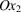:There are 8 independent components. (ii) Group m:There are 10 independent components. (iii) Group: all the components are equal to zero.

#### 1.1.4.10.4.3. Orthorhombic system

| top | pdf |

 (i) Group 222:There are 3 independent components. (ii) Group:There are 5 independent components. (iii) Group: all the components are equal to zero.

#### 1.1.4.10.4.4. Trigonal system

| top | pdf |

 (i) Group 3:where the symbol ⊖ means that the corresponding component is equal to the opposite of that to which it is linked, ⊙ means that the component is equal to twice minus the value of the component to which it is linked for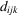and to minus the value of the component to which it is linked for. There are 6 independent components. (ii) Group 32, twofold axis parallel to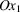:with the same conventions. There are 4 independent components. (iii) Group, mirror perpendicular to:with the same conventions. There are 4 independent components. (iv) Groupsand: all the components are equal to zero.

#### 1.1.4.10.4.5. Tetragonal, hexagonal and cylindrical systems

| top | pdf |

 (i) Groups 4, 6 and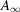:There are 4 independent components. (ii) Groups 422, 622 and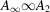:There is 1 independent component. (iii) Groups,and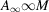:There are 3 independent components. (iv) Groups,and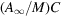: all the components are equal to zero. (v) Group:There are 4 independent components. (vi) Group:with the same conventions as for group 3. There are 2 independent components. (vii) Group– twofold axis parallel to:There are 2 independent components. (viii) Group– mirror perpendicular to(twofold axis at):The number of independent components is of course the same. (ix) Group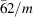:with the same conventions as for group 3. There is 1 independent component. (x) Groups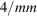,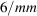and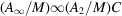: all the components are equal to zero.

#### 1.1.4.10.4.6. Cubic and spherical systems

| top | pdf |

 (i) Groups 23 and:There is 1 independent component. (ii) Groups 432 and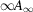: it was seen in Section 1.1.4.8.6that we have in this case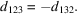It follows that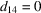, all the components are equal to zero. (iii) Groups,and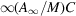: all the components are equal to zero.